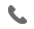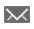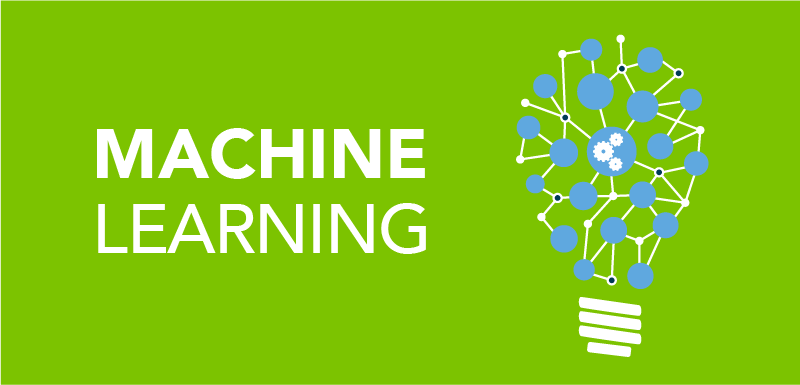•+44 7459 302492info@uplatz.com
• Register
Job Meter = High

### Career Path - Artificial Intelligence and Machine Learning Engineer

50 Hours
Online Instructor-led Training
USD 2800Artificial Intelligence (AI) is a computer system’s ability to mimic human behavior. Machines demonstrate this sort of intelligence, which can be compared to a natural intelligence that humans and animals demonstrate. An AI engineer builds AI models using machine learning algorithms and deep learning neural networks to draw business insights, which can be used to make business decisions that affect the entire organization. These engineers also create weak or strong AIs, depending on what goals they want to achieve.

Machine learning (ML) is a type of artificial intelligence that allows software applications to become more accurate at predicting outcomes without being explicitly programmed to do so. Machine learning algorithms use historical data as input to predict new output values.
This Aritificial Intelligence / Machine Learning Career Path program by Uplatz will help you acquire skills necessary to become a successful Machine Learning / Deep Learning Engineer. This can be a game-changer for your career and can put you on track for a highly-paid lucrative and challenging career in cutting-edge technology.

# Career Path - Artificial Intelligence and Machine Learning Engineer

Course Details & Curriculum

Machine Learning Basics –Course Syllabus

Introduction:

1.    Getting Started with Machine Learning

2.    An Introduction to Machine Learning

3.    What is Machine Learning?

4.    Introduction to Data in Machine Learning

5.    Demystifying Machine Learning

6.    ML – Applications

7.    Best Python libraries for Machine Learning

8.    Artificial Intelligence | An Introduction

9.    Machine Learning and Artificial Intelligence

10. Difference between Machine learning and Artificial Intelligence

11. Agents in Artificial Intelligence

12. 10 Basic Machine Learning Interview Questions

Data and It’s Processing:

1.    Introduction to Data in Machine Learning

2.    Understanding Data Processing

3.    Python | Create Test DataSets using Sklearn

4.    Python | Generate test datasets for Machine learning

5.    Python | Data Preprocessing in Python

6.    Data Cleansing

7.    Feature Scaling – Part 1

8.    Feature Scaling – Part 2

9.    Python | Label Encoding of datasets

10. Python | One Hot Encoding of datasets

11. Handling Imbalanced Data with SMOTE and Near Miss Algorithm in Python

Supervised learning :

1.    Getting started with Classification

2.    Basic Concept of Classification

3.    Types of Regression Techniques

4.    Classification vs Regression

5.    ML | Types of Learning – Supervised Learning

6.    Multiclass classification using scikit-learn

·        Gradient Descent algorithm and its variants

·        Mini-Batch Gradient Descent with Python

·        Optimization techniques for Gradient Descent

·        Introduction to Momentum-based Gradient Optimizer

8.    Linear Regression:

·        Introduction to Linear Regression

·        Gradient Descent in Linear Regression

·        Mathematical explanation for Linear Regression working

·        Normal Equation in Linear Regression

·        Linear Regression (Python Implementation)

·        Simple Linear-Regression using R

·        Univariate Linear Regression in Python

·        Multiple Linear Regression using Python

·        Multiple Linear Regression using R

·        Locally weighted Linear Regression

·        Python | Linear Regression using sklearn

·        Linear Regression Using Tensorflow

·        A Practical approach to Simple Linear Regression using R

·        Linear Regression using PyTorch

·        Pyspark | Linear regression using Apache MLlib

·        ML | Boston Housing Kaggle Challenge with Linear Regression

9.    Python | Implementation of Polynomial Regression

10. Softmax Regression using TensorFlow

11. Logistic Regression:

·        Understanding Logistic Regression

·        Why Logistic Regression in Classification?

·        Logistic Regression using Python

·        Cost function in Logistic Regression

·        Logistic Regression using Tensorflow

12. Naive Bayes Classifiers

13. Support Vector:

·        Support Vector Machines(SVMs) in Python

·        SVM Hyperparameter Tuning using GridSearchCV

·        Support Vector Machines(SVMs) in R

·        Using SVM to perform classification on a non-linear dataset

14. Decision Tree:

·        Decision Tree

·        Decision Tree Regression using sklearn

·        Decision Tree Introduction with example

·        Decision tree implementation using Python

·        Decision Tree in Software Engineering

Certification
Course Completion Certificate

The Course completion certificate will be awarded by Uplatz.

Career Path

The main objective of this course is to introduce the basic techniques, principles and applications of artificial intelligence and machine learning. Specifically:

To gain an in-depth perspective of AI and its foundations

To provide a strong foundation of fundamental concepts in Machine learning and Artificial Intelligence

To provide a basic exposition to the methods of Artificial Intelligence

To make use of different machine learning techniques to design AI machinesfor solving real-world problems

Have solid programming skills and know Python, R, Go, Java, SQL

Understand how TensorFlow and other similar technologies work

Have a clear understanding about technologies related to AI, including Machine Learning, Neural Networks and Deep Learning